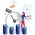## 21 September, 2015

### The Posterior Predictive

Let's say you do a Bayesian analysis and end up with a posterior distribution $p(\theta | y)$. What does that tell you about a new observation $\tilde{y}$ from some data-generating process that involves $\theta$? The answer can be found using the posterior predictive distribution.

The code that I used to generate the images in this article can be found on my github.

## Posterior Predictive

Given a posterior distribution $p(\theta | y)$, the posterior predictive distribution is defined as

$p(\tilde{y} | y) = \int p(\tilde{y} | \theta) p(\theta | y) d\theta$

and it represents the distribution of a new observation $\tilde{y}$ given your updated information about a parameter $\theta$ and natural variation around the observation that arises from the data-generating process.

Since applied Bayesian techniques have tended towards fully computational MCMC procedures, the posterior predictive is usually obtained through simulation - let's say you have a sample $\theta_j^*$ ($j = 1,2,...,k$) from the posterior distribution of $\theta$ and you want to know about a new observation from some process that uses the parameter $\theta$.

$\tilde{y} \sim p(\tilde{y} | \theta)$

To obtain the posterior predictive, you would simulate a set of observation $\tilde{y}^*_j$ from $p(\tilde{y} | \theta_j^*)$ (in other words, simulate a new observation from the data model for each draw from the MCMC for your parameter). The distribution of these  $\tilde{y}^*_j$ approximates the posterior predictive distribution for $\tilde{y}$.

In general, this process is very specific to the problem at hand. It is possible in a few common scenarios, however, to calculate the posterior predictive distribution analytically. One example that is useful in baseball analysis the beta-binomial model.

## Beta-Binomial Example

Let's say a batter obtains a 0.300 OBP in 250 PA - that corresponds to 75 on-base events and 175 not on-base events. What can you say about the distribution of on-base events in a new set of 250 PA?

Suppose that the distribution of on-base events is given by a binomial distribution with $n = 250$ and chance of getting on base $\theta$, which is the same in both sets of PA.

$p(y | \theta) \sim Binomial(250, \theta)$

For the prior distribution, let's suppose that a $Beta(1,1)$ distribution was used - this is a uniform distribution between zero and one - so any possible value for $\theta$ is equally likely. Since the beta and binomial are conjugate distributions, the posterior distribution of  $\theta$ (the batter's chance of getting on base) is also a beta distribution:

$p(\theta| y = 75) = \dfrac{\theta^{75+1-1}(1-\theta)^{175+1-1}}{\beta(75+1,175+1)} \sim Beta(76,176)$

Now, suppose we are planning to observe another 250 PA for the same batter, and we want to know the distribution of on-base events $\tilde{y}$ in the new 250 PA. This distribution is also binomial

$p(\tilde{y} | \theta) = \displaystyle {250 \choose \tilde{y}} \theta^{\tilde{y}}(1-\theta)^{250-\tilde{y}}$

The posterior predictive distribution for the number of on-base events in another 250 PA is then obtained by multiplying the two densities and integrating out $\theta$.

$p(\tilde{y} | y = 75) = \displaystyle \int_0^1 {250 \choose \tilde{y}} \theta^{\tilde{y}}(1-\theta)^{250-\tilde{y}} * \dfrac{\theta^{75}(1-\theta)^{175}}{\beta(76,176)} d\theta$

The resulting distribution is known as beta-binomial distribution, which has density

$p(\tilde{y} | y = 75) =\displaystyle {250 \choose \tilde{y}} \dfrac{\beta(76 + \tilde{y}, 426-\tilde{y})}{\beta(76,176)}$

(The beta-binomial distribution is obtained from the beta-binomial model - it does get a bit confusing, but they are different things - the beta-binomial model can be thought of as a binomial with extra variance)

Now I can use the posterior predictive for inference. If, for example, I wanted to know the probability that a player will have a 0.300 OBP in another 250 PA (corresponding, again, to $\tilde{y}$ = 75 on-base events) then I can calculate that as

$p(\tilde{y} = 75 | y = 75) = \displaystyle {250 \choose 75} \dfrac{\beta(76 + 75, 426-75)}{\beta(76,176)} \approx 0.0389$

That is, our updated information says there's a 3.89% chance of getting exactly a 0.300 OBP in a new 250 PA by the same player.

The actual distribution of OBP in a new 250 PA is given by

This can also be accomplished by simulation - first, by simulating a large number $k$ of $\theta^*$ values from the posterior

$\theta^*_j \sim Beta(76,176)$

And then using those $\theta^*$ values to simulate from the data model $p(\tilde{y} | \theta^*)$

$y^*_j \sim Binomial(250, \theta^*_j)$

The estimated probability of a 0.300 OBP (equivalent to 75 on-base events) is then

$P(0.300 | y = 75) \approx \displaystyle \dfrac{\textrm{# } y^*_j \textrm{ that equal 75}}{k}$

This is much easier to do in $R$ -with $k = 1000000$, the code to quickly perform this is

> theta <- rbeta(1000000, 76,176) #Simulate from Posterior
> y <- rbinom(1000000, 250, theta) #Simulate from data model
> mean(y == 75) #Estimate P(y = 75)
 0.039077

Notice that the result is very close to the analytic answer of 3.89%. The simulated posterior predictive distribution for OBP is

Which visually looks very similar to the analytic version.

## What's the Use?

Aside from doing (as the name implies) prediction, the posterior predictive is very useful for model checking - if data simulated from the posterior predictive (new data) is similar to the data you used to fit the model (old data), that is evidence that the model is a good fit. Conversely, if your posterior predictive data looks nothing like your original data set, you may have misspecified your model (and I'm criminally oversimplifying here - I recommend Bayesian Data Analysis by Gelman et al. for all the detail on model-checking using the posterior predictive distribution).

#### 1 comment:

1.u can do it with pbetap funtion in R with LeanrBayes package and I think we may also caculate it with Gibbs Sampling and why everyone just visit this topic in 2020 lol